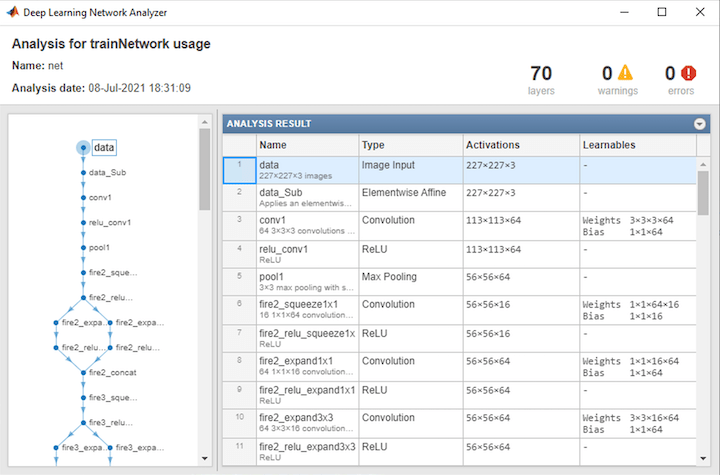# importONNXNetwork

Import pretrained ONNX network

## Syntax

``net = importONNXNetwork(modelfile,'OutputLayerType',outputtype)``
``net = importONNXNetwork(modelfile,'OutputLayerType',outputtype,'Classes',classes)``

## Description

````net = importONNXNetwork(modelfile,'OutputLayerType',outputtype)` imports a pretrained network from the ONNX™ (Open Neural Network Exchange) file `modelfile` and specifies the output layer type of the imported network.This function requires the Deep Learning Toolbox™ Converter for ONNX Model Format support package. If this support package is not installed, then the function provides a download link.```

example

````net = importONNXNetwork(modelfile,'OutputLayerType',outputtype,'Classes',classes)` additionally specifies the classes for a classification network.```

## Examples

collapse all

Download and install the Deep Learning Toolbox Converter for ONNX Model Format support package.

Type `importONNXNetwork` at the command line.

`importONNXNetwork`

If Deep Learning Toolbox Converter for ONNX Model Format is not installed, then the function provides a link to the required support package in the Add-On Explorer. To install the support package, click the link, and then click . Check that the installation is successful by importing the network from the model file `'cifarResNet.onnx'` at the command line. If the support package is installed, then the function returns a `DAGNetwork` object.

```modelfile = 'cifarResNet.onnx'; classes = ["airplane" "automobile" "bird" "cat" "dee" "dog" "frog" "horse" "ship" "truck"]; net = importONNXNetwork(modelfile,'OutputLayerType','classification','Classes',classes)```
```net = DAGNetwork with properties: Layers: [77×1 nnet.cnn.layer.Layer] Connections: [85×2 table]```

Import a residual neural network trained on the CIFAR-10 data set. Specify the file containing the ONNX network, its output type, and its output classes.

```modelfile = 'cifarResNet.onnx'; classes = ["airplane" "automobile" "bird" "cat" "deer" "dog" "frog" "horse" "ship" "truck"]; net = importONNXNetwork(modelfile,'OutputLayerType','classification','Classes',classes)```
```net = DAGNetwork with properties: Layers: [77×1 nnet.cnn.layer.Layer] Connections: [85×2 table] InputNames: {'Input_input'} OutputNames: {'ClassificationLayer_softmax'} ```

Analyze the imported network.

`analyzeNetwork(net)`## Input Arguments

collapse all

Name of ONNX model file containing the network, specified as a character vector or a string scalar. The file must be in the current folder, in a folder on the MATLAB® path, or you must include a full or relative path to the file.

Example: `'cifarResNet.onnx'`

Type of the output layer that the function appends to the end of the imported network, specified as `'classification'`, `'regression'`, or `'pixelclassification'`. Using `'pixelclassification'` appends a `pixelClassificationLayer` (Computer Vision Toolbox) object (requires Computer Vision Toolbox™).

If a network in `modelfile` has multiple outputs, then you cannot specify the output layer types using this argument. Use `importONNXLayers` instead. `importONNXLayers` inserts placeholder layers for the outputs. After importing, you can find and replace the placeholder layers by using `findPlaceholderLayers` and `replaceLayer`, respectively.

Example: `'regression'`

Classes of the output layer, specified as a categorical vector, string array, cell array of character vectors, or `'auto'`. If `Classes` is `'auto'`, then the software sets the classes to `categorical(1:N)`, where `N` is the number of classes. If you specify a string array or cell array of character vectors `str`, then the software sets the classes of the output layer to `categorical(str,str)`.

Data Types: `char` | `categorical` | `string` | `cell`

## Output Arguments

collapse all

Pretrained network, returned as `DAGNetwork` object.

## Limitations

• `importONNXNetwork` supports ONNX versions as follows:

• `importONNXNetwork` supports ONNX intermediate representation version 6.

• `importONNXNetwork` fully supports ONNX operator sets 6, 7, 8, and 9.

• `importONNXNetwork` offers limited support for ONNX operator sets 10 and 11.

Note

If you import an exported network, layers of the reimported network might differ from the original network and might not be supported.

## Compatibility Considerations

expand all

Not recommended starting in R2018b

 Open Neural Network Exchange. https://github.com/onnx/.

 ONNX. https://onnx.ai/.